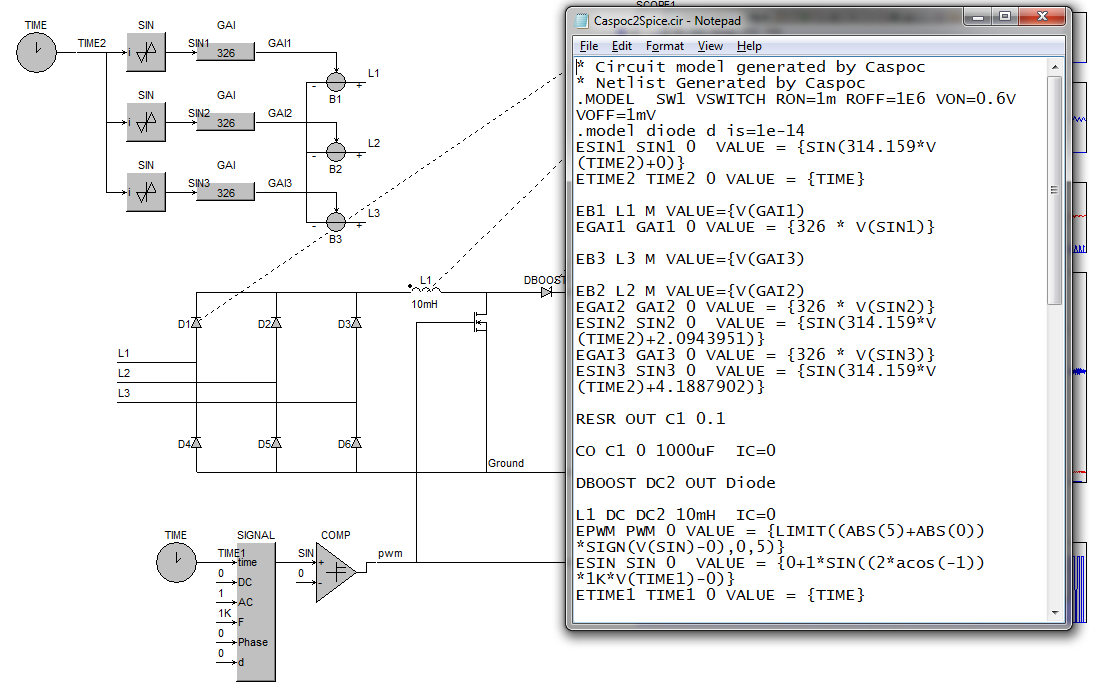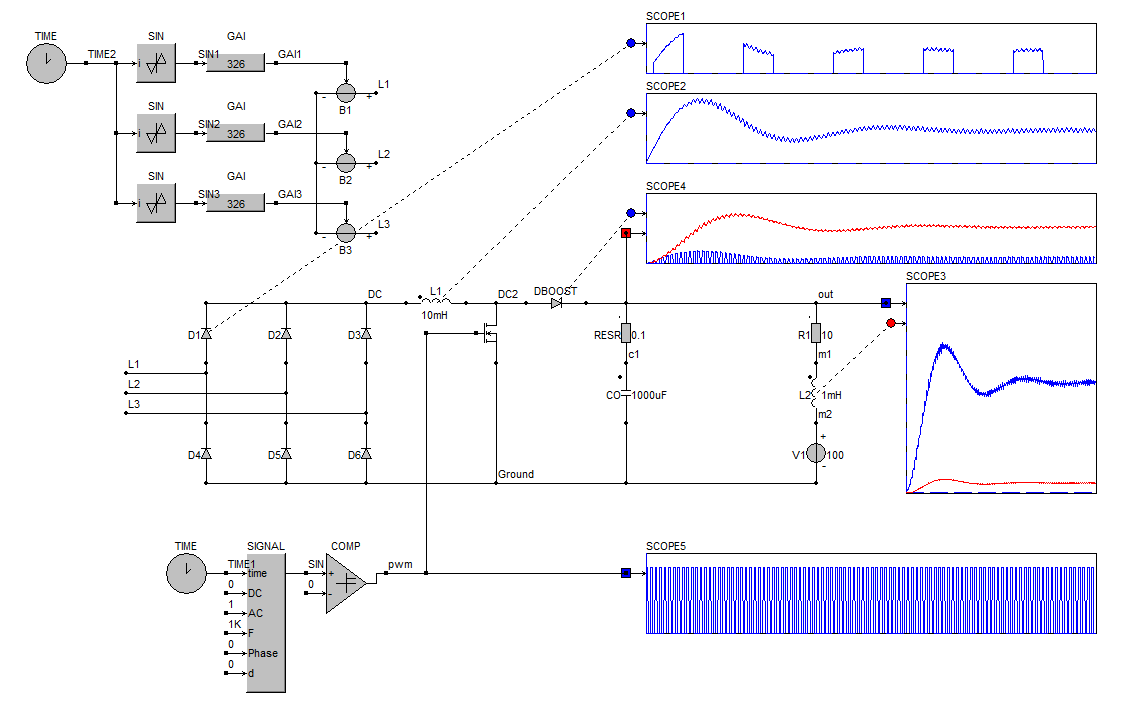• Introduction
• What is in this manual
• What is Caspoc
• User interface
• Introduction
• Starting
• Simulation
• Editing
• Viewing and printing
• Getting Started
• Basic editing
• Simulation in the time domain
• Basic User Interface Topics
• Editing
• Simulation
• Viewing
• Library
• Reports
• Project management
• Circuit and Block Diagram Components
• Introduction
• Cscript and user defined functions
• Component parameters
• Modeling Topics
• Introduction
• Power Electronics
• Semiconductors
• Electrical Machines
• Electrical drives
• Power Systems
• Mechanical Systems
• Thermal Systems
• Magnetic Circuits
• Green Energy
• Coupling to FEM
• Experimenter
• Analog hardware description language
• Embedded C code Export
• Coupling to Spice
• Small Signal Analysis
• Matlab coupling
• Tips and tricks
• Appendices

## Export Caspoc models into a Spice netlist.In this section we will export a complete Caspoc model into a Spice netlist

Most of the syntax of the Caspoc circuit models and the spice models are equal. Thies means that connecting resistors, capacitors, inductors and current and voltage sources is equal in syntax. There are some extra components that will only work in Caspoc, as they require a special simulator kernel.

The other main difference is the way the electric circuit is simulated in Caspoc. In contrary to the spice simulators, Caspoc uses a special algorithm for solving the circuit simulations containing switches such as diodes and mosfets. Secondly it can handle inductors in series, which is impossible in Spice. For this reason care has to be taken when performing spice simulations and comparing the results with Caspoc.

In Caspoc you can use the ideal diode model for simulating rectifier circuits. The switching of the diodes is determined by the input voltages and the DC voltage at the output. The ideal diode models are either on or off. In Spice there is a diode model, but it follows a non-linear V_I relation. This makes sense in electronic circuit simulation, but in the case of rectifier simulations, where the diode is either turned on or turned off.

Another difference is the way control systems are modelled. In Caspoc there are block diagram components that have inputs and an output. These components only process analog signals and not voltage-current relations. Because of that simulations in Caspoc are generally faster than in Spice.

Exporting the block diagram components into a spice netlist results in a lumped circuit with controlled sources representing the control structure of the block diagram.

### Three phase inverter with boost converter

The schematic below is a Caspoc simulation of a three phase rectifier connected to three controlled voltage sources. The output from the rectifier is connected to a boost converter operating with a constant duty-cycle.After running the simulation, the results are displayed in the scopes. This shows that the model contains all components and is configured in the correct way. To export the spice netlist, you have to select from the menu Tools/Spice/Export and than select the preferred file type. Below we are exporting as a spice *.cir file.The spice netlist file is written in the temporary folder of Windows. To see the results, you have to select the menu item Tools/Spice/View/View (exported) input files and than select the preferred file typeOnly after selectingview from the menu, the netlist will be shown in the preferred text editor.